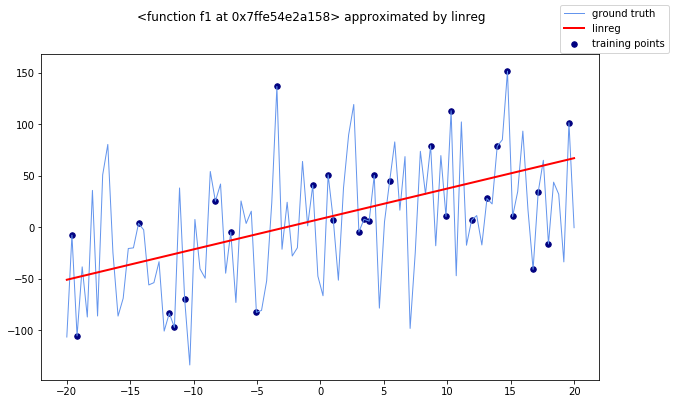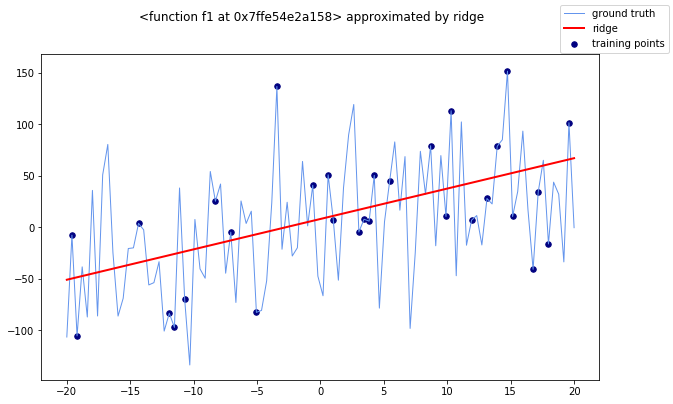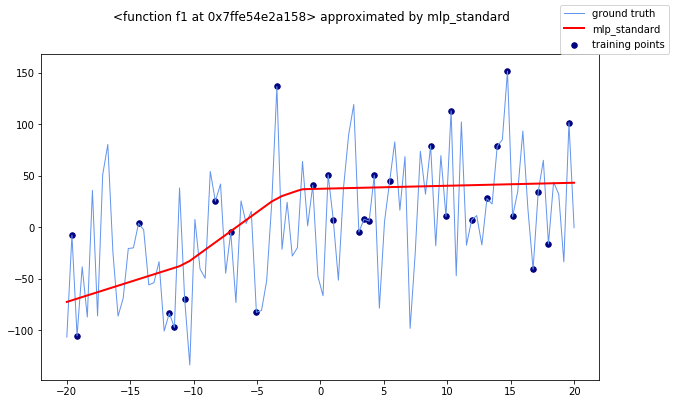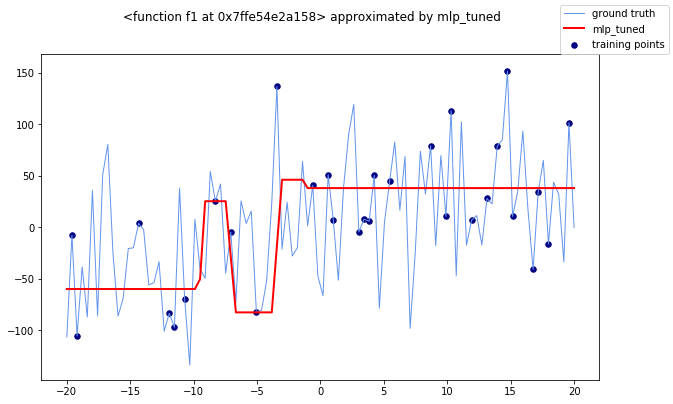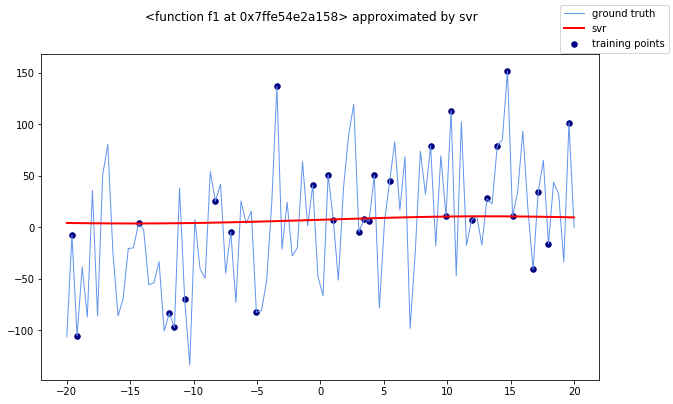In :
import numpy as np
import pandas as pd
import matplotlib.pyplot as plt
%matplotlib inline

In :
plt.rcParams["figure.figsize"] = (10, 6)


## Exercise 1¶

In this exercise, we will try different regression models on points drawn from three different analytical functions. We will see that the overall quality of the models heavily depends on the shape of the functions.

That said, let's define our three analytical functions.

In :
def f1(x):
return x * np.sin(x) + 2*x

def f2(x):
return 10 * np.sin(x) + x**2

def f3(x):
return np.sign(x) * (300 + x**2) + 20 * np.sin(x)


### Exercises 1.1 and 1.2¶

As usual, we can define convenient functions to generate and plot our data.

In :
def generate_X_y(f):
tr = 20
n_samples = 100
X = np.linspace(-tr, tr, n_samples)
y = f(X)
return X, y

def plot_f(X, y, title):
LW = 4
fig, ax = plt.subplots()
ax.plot(X, y, color='cornflowerblue', linewidth=.5*LW, label="ground truth")
fig.suptitle(title)
#ax.scatter(X_train, y_train, color='navy', s=30, marker='o', label="training points")

In :
for f in [f1, f2, f3]:
X, y = generate_X_y(f)
plot_f(X, y, f)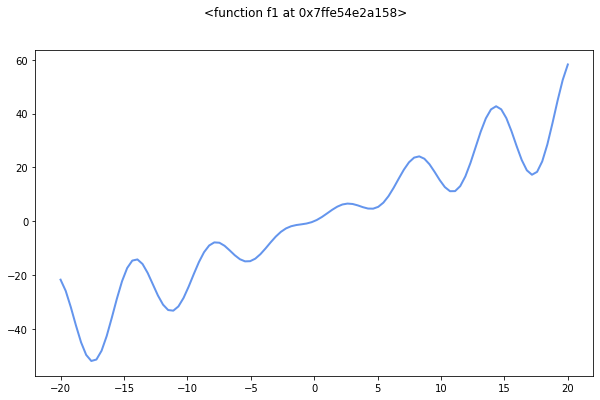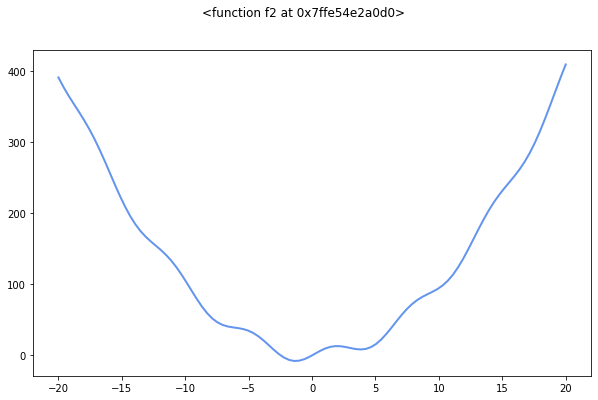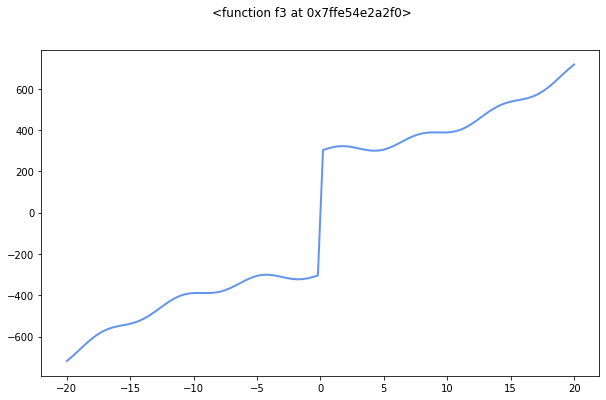f1 has two main components: a sine wave with an increasing magnitude (due to the factor x), plus an additive factor that gives a non zero slope to the curve.

f2 has a sine wave with fixed amplitude, modulated by a parabolic function.

f3 presents a sine wave modulated by a parabolic function. However, it is different from f2. The quadratic expression changes concativity (i.e. there is an inflection point) at zero, due to the sign component. Also, the factor sign(x) 300 produces a discontinuity point of type one.

The shape of the functions tells us that f2 and f3 have an infinite asymptotic value, for both positive and negative values. Hence, they can be approximated with polynomial regressors. However, f3 has a discontinuity point at 0, which can harden the approximation for classifiers. Even if the ordinary least squares regressor would fit the linear trend present in f1, we can see that all the three functions cannot be approximated with a linear regressor, with sufficient results, for large values of x.

Back to programming, you can notice how the functions behave as simple objects in Python. It is not unsual to assign them to variables or, like in this case, create an array of them. Here, the f variable points, at each loop cycle, to a different function in memory, and gets invoked as a callable object. Additionally, you can note the printed version of f contains the name of the assigned function and the memory address where its code is stored.

#### Exercises 1.3, 1.4, and 1.5¶

Let's now fit our regression models. To do so, we define a function to create the training and test points given a function and a scikit-learn Pipeline to apply to them. For the seek of readability, we inspect one analytic function at a time.

In :
from sklearn.model_selection import train_test_split

def generate_train_test(f, X, y):
X_train, X_test, y_train, y_test = train_test_split(X, y,
train_size=30,
random_state=42,
shuffle=True)
y_test = y_test[X_test.argsort()]
X_test.sort()
return X_train, X_test, y_train, y_test


Note that here the cardinality of training and test sets are reversed with respect to common cases. Typically, the 70% of the dataset is kept as training set and the remaining 30% is used to test the model.

In the following cells, we test different regression algorithm and evaluate the regression error through two metrics: the MSE and the R2 score. Thus, we use a convenient Python function that, given an analytical function, a dataset, and a model, produces the value of the two metrics. To better understand the quality of each regressor, the function provides also a graphical representation of the predicted values against the real values drawn from the original curve.

In :
from sklearn.metrics import mean_squared_error
from sklearn.metrics import r2_score

def evaluate_model(f, X, y, model, model_name):
X_train, X_test, y_train, y_test = generate_train_test(f, X, y)

# plot the real function and the training points
LW = 2
fig, ax = plt.subplots()
ax.plot(X, y, color='cornflowerblue', linewidth=.5*LW, label="ground truth")
ax.scatter(X_train, y_train, color='navy', s=30, marker='o', label="training points")

# predict the test points and plot them onto the chart
model.fit(X_train.reshape(-1, 1), y_train)
y_hat = model.predict(X_test.reshape(-1, 1))
ax.plot(X_test, y_hat, linewidth=LW, label=name, color='r')

fig.suptitle(f"{f} approximated by {model_name}")
fig.legend()

return mean_squared_error(y_test, y_hat), r2_score(y_test, y_hat)

In :
from sklearn.linear_model import LinearRegression
from sklearn.neural_network import MLPRegressor
from sklearn.svm import SVR
from sklearn.ensemble import RandomForestRegressor
from sklearn.linear_model import Ridge
from sklearn.preprocessing import FunctionTransformer, PolynomialFeatures
from sklearn.compose import make_column_transformer
from sklearn.pipeline import make_pipeline


The performance of each regressor are collected and displayed with the library PrettyTable.

In :
from prettytable import PrettyTable

degree = 5
models = [
LinearRegression(),
Ridge(random_state=42),
MLPRegressor(hidden_layer_sizes=(10,), random_state=42, max_iter=10000),
MLPRegressor(hidden_layer_sizes=(10,10),  activation='tanh', solver='lbfgs',
alpha=0.000, batch_size='auto', learning_rate='constant',
learning_rate_init=0.01, power_t=0.5, max_iter=10000, shuffle=True,
random_state=42, tol=0.0001, verbose=True, warm_start=False,
momentum=0.0, nesterovs_momentum=False, early_stopping=False,
validation_fraction=0.0, beta_1=0.9, beta_2=0.999, epsilon=1e-08),
SVR(gamma='scale'),
RandomForestRegressor(n_estimators=300),
make_pipeline(
make_column_transformer(
(FunctionTransformer(np.sin), ),
(PolynomialFeatures(degree), )
),
LinearRegression()
),
make_pipeline(
make_column_transformer(
(FunctionTransformer(np.sin), ),
(PolynomialFeatures(degree), )
),
Ridge(alpha=1)
)
]

names = [
'linreg',
'ridge',
'mlp_standard',
'mlp_tuned',
'svr',
'rf',
f'sin+poly{degree}+linreg',
f'sin+poly{degree}+ridge'
]


Before the actual simulation, let's spend a few comments on the code above. We generated a list with a few models to be tested along with a respective textual representation that is used in the title of the each figure. The single-stage models are:

• a simple Linear Regression model;
• a MultiLayer Perceptron (a.k.a. feed forward neural network) with a single hidden layer with a reasonable number of hidden nodes;
• a deeper MultiLayer Perceptron with tuned parameters. We do not spend too much time on them since it goes beyond the scope of the exercise. However, the provided paramenters should let you grasp how wide are the tuning possibilities of this estimator;
• a Support Vector Regressor (gamma = 'scale' is recommended for the scikit-learn implementation);
• a Random Forest Regressor (the higher the number of estimator, the better).

We also adopted two composite pipelines. Each of them has two steps:

1. in the first step we build a ColumnTransformer using an utility method. The Transform objects in scikit-learn's preprocessing module are typically used to perfom some sort of transformation of the input columns (e.g. scaling and normalization, application of a function). The ColumnTransform lets you specify a series of single Transformers to apply to columns of your choice. In our case, we apply two types of column transformation to the first (and only) column (see the parameter  of each tuple). One uses a FunctionTransformer to generate one additional feature in the form sin(x). The other creates new polynomial features using the pattern provided by the class PolynomialFeatures.
2. In the second test we apply the regressor model as usual. Specifically, we test LinearRegression a Ridge again to measure the impact of the previous preprocessing on the performance.

#### Results for f = f1¶

In :
t = PrettyTable()
t.field_names = ['model', 'MSE', 'R2']
X, y = generate_X_y(f1)
for model, name in zip(models, names):
mse, r2 = evaluate_model(f1, X, y, model, name)
t.add_row([name, mse, r2])

print(t)

+------------------+--------------------+---------------------+
|      model       |        MSE         |          R2         |
+------------------+--------------------+---------------------+
|      linreg      | 65.72227592689515  |  0.8950661957810067 |
|      ridge       | 65.74765554283465  |  0.8950256740612001 |
|   mlp_standard   | 75.46463406818695  |  0.879511306857702  |
|    mlp_tuned     | 104.46617412806707 |  0.8332067338073432 |
|       svr        | 399.9552743551347  | 0.36142155968215894 |
|        rf        | 57.024236887693924 |  0.9089536991085534 |
| sin+poly5+linreg | 95.50251981840506  |  0.8475183250167385 |
| sin+poly5+ridge  | 93.41072390557375  |  0.8508581378836467 |
+------------------+--------------------+---------------------+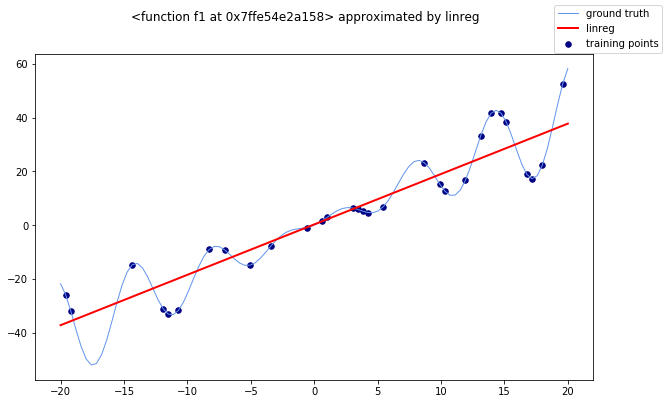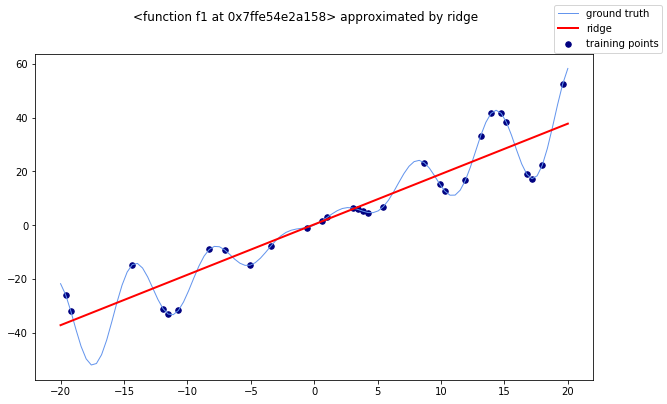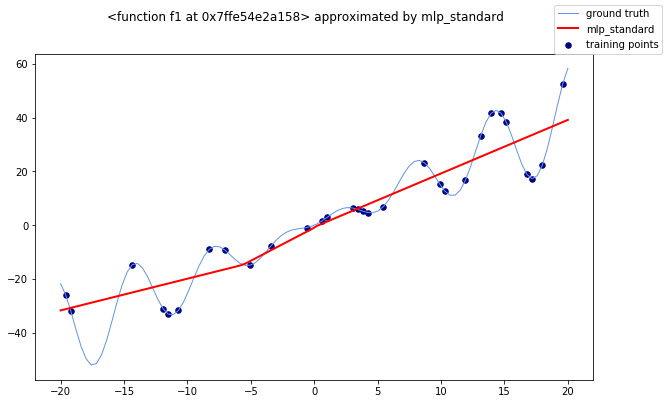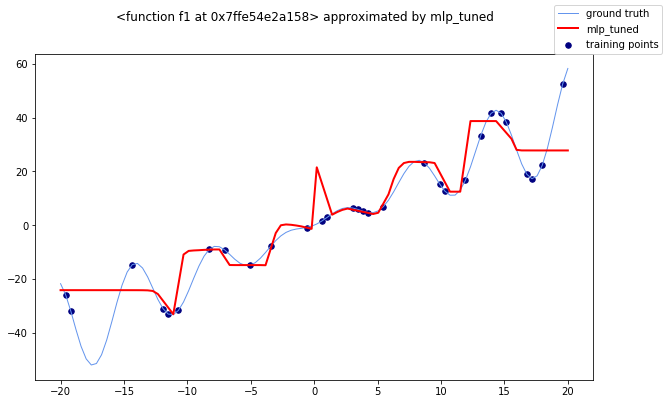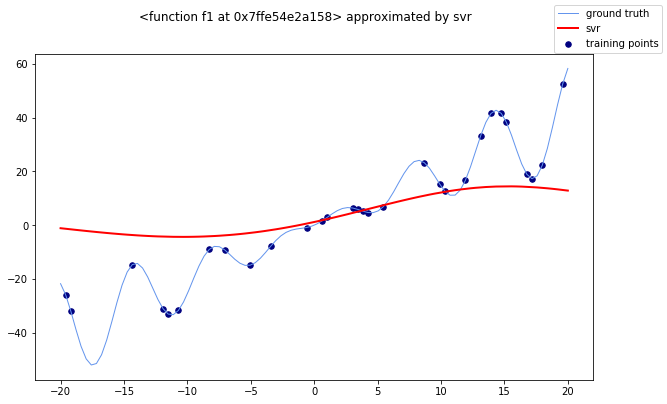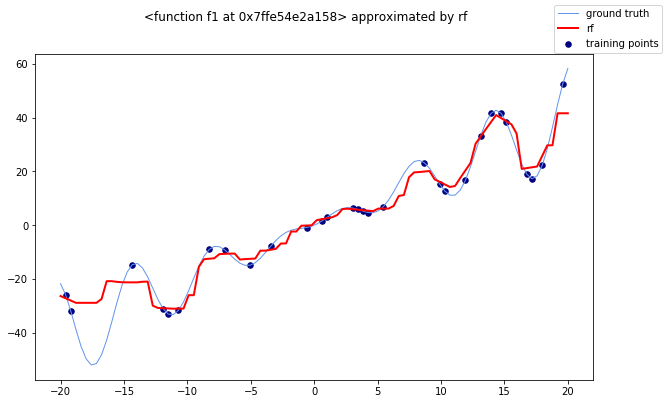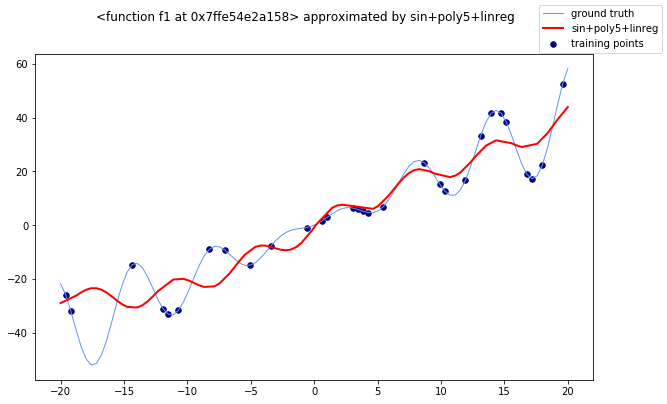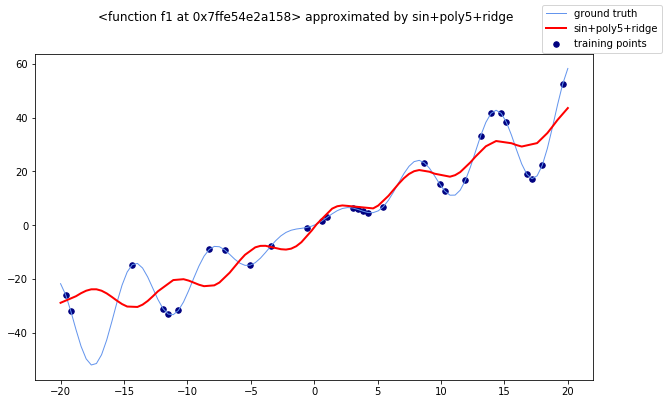We see that LinearRegression and Ridge have comparable results. SVR fails at modeling the correct shape of the curve, the standard MLP converged to an approximation of the linear behavior, while the tuned MLP shows a more complex pattern, although an abnormal spike near zero worsen probably affects negatively the performance. The Random Forest regressor achieved the best performance in both the MSE and R2 scores. Finally, it is worth noting that the addition of sin(x) and the polynomial features up to the fifth degree slightly worsened the performance of LinearRegression and Ridge. Having the real function shape, we can graphically find a motivation for that. For both the regressors, the predictions (the red curve) are able follow a sinusoidal pattern (which we might have expected by the introduction of sin(x)). Nonetheless, for negative values of x, this curve is in couterphase with the real one, increasing the overall error.

### Exercise 1.6¶

In many real tasks, we typically suppose that the measurements of the predictive variables carry some sort of noise. To reflect this aspect to our synthetic data, we can inject it manually.

In :
def inject_noise(x):
"""Add a random noise drawn from a normal distribution."""
return x + np.random.normal(0, 50, size=x.size)

In :
X, y = generate_X_y(f1)
y = inject_noise(y)

t = PrettyTable()
t.field_names = ['model', 'MSE', 'R2']
for model, name in zip(models, names):
mse, r2 = evaluate_model(f1, X, y, model, name)
t.add_row([name, mse, r2])

print(t)

/Users/giuseppe/miniconda3/lib/python3.6/site-packages/sklearn/neural_network/_multilayer_perceptron.py:571: ConvergenceWarning: Stochastic Optimizer: Maximum iterations (10000) reached and the optimization hasn't converged yet.
% self.max_iter, ConvergenceWarning)

+------------------+--------------------+----------------------+
|      model       |        MSE         |          R2          |
+------------------+--------------------+----------------------+
|      linreg      | 2957.236994778774  | 0.11280320127342303  |
|      ridge       | 2957.0994988641382 | 0.11284445124274178  |
|   mlp_standard   | 3296.3441666075573 | 0.011068102665194646 |
|    mlp_tuned     | 3688.7875228403523 | -0.10666831479001027 |
|       svr        | 3324.973510100103  | 0.002479050810008321 |
|        rf        | 4556.727445167173  | -0.36705783444464113 |
| sin+poly5+linreg | 3288.756662982372  | 0.013344419693040566 |
| sin+poly5+ridge  | 3289.5728746771806 | 0.01309954909119293  |
+------------------+--------------------+----------------------+